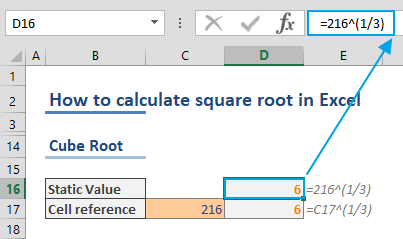The square root of a number is a value which is equal to the number, when multiplied by itself. In this guide, we're going to show you how to find square root in Excel using two different methods.

## How to find square root using the SQRT function

Excel's SQRT function can calculate and return the square root of a specified number. The function is very easy-to-use and only requires a single argument - the number for which you want to find the square root.

Let's see how the function works on an example. To calculate the square root of 25, you can use a function like the following:

=SQRT(25)## "Manual" square root calculation

To calculate the square root of a number "manually", you can use the exponential version instead (i.e. 251/2). You can apply this same idea in Excel using a formula like below.

=25^(1/2)The caret symbol (^) in the formula represents the exponentiation operation. For example, 5 ^ 2 equals 25. On the other hand, using a fraction after the caret symbol (^) represents rational exponents.

In the light of this information, we can calculate the nth root of a number by placing n into rational exponent. For example;

Remember the parenthesis around the fraction. Otherwise, Excel evaluate x^1 first and then executes the division operation.

## Calculating cube root of a number

After understanding the logic behind rational exponents, calculating the cube or nth root of a number is just as easy. Also, keep in mind that Excel doesn't have a dedicated function to calculate the cube root.

This application is the same as described in the "manual" method: enter an n value into the fraction to find the nth root of a specified number. For the cube root calculation, you need to set n as 3. Thus, the formula to find the cube root of 216 would be something like below.

=216^(1/3)